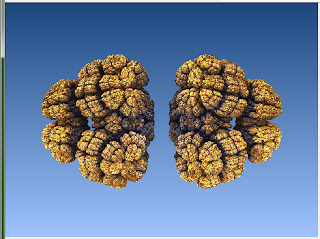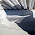## Saturday, September 24, 2011

### Mandelbulb 3D Tutorial: Julia Mode

Ok, back once again for another M3D tutorial page!

I'm going to assume that most of you already know what a Julia fractal is. (Not that it is vital for you to be able to use the Julia mode tab in M3D ... But if you're one of those people who dove straight into M3D and 3D fractals because of how cool they look, and understand little else about Mandelbrot, or Julia, etc, go have a look at my Introduction to Fractals tutorial. It will probably be worth it :) ).

Ok, well, in M3D, just as 2D fractals are plotted out on the complex plane (cartesian x-y axis), 3D fractals are plotted out in a cartesian complex "space" (x,y and z .. There's a "w" too sometimes but I'm really not sure how that works (yet!)).

And just as every point on that complex plane has a corresponding Julia, so does every infinite "voxel" in the 3D complex space.

Don't worry if you don't understand this... Just go ahead and try it out. I loaded up the first formula on the list: Benesi1Pow2.

Then, I simply clicked on the Julia tab, checked the little "Calculate Julia" tick box (with x=1, the rest 0), and clicked the Calculate 3D button.

Not a very interesting fractal I know... But I then played around with the x,y and z co-ordinates, and here's what I got:Benesi1Pow2Julia x=0.1 y=0.4 z=0.2Benesi1Pow2Julia x=0 y=1 z=0Benesi1Pow2Julia x=-0.2 y=-0.1 z=0Benesi1Pow2Julia x=0.5 y=0.5 z=0.5Benesi1Pow2Julia x=-1 y=0.8 z=-0.4Benesi1Pow2 Julia x=0 y=0 z=1
As you can see, a lot of variation is possible with only one formula. And if you're zoomed into a Julia quite deeply, you can try tweaking one of the x,y or z parameters only very slightly, to see what changes :) ... It's a matter of trial and error, but some amazing images can be found...

My Fractal Worlds that I've just started (see previous blog entries) were all discovered using Julias of various hybrid formulae. (That's something to remember: You can combine different formulae and then apply the Julia, or you can choose one formulae, find a great julia, and then fuse it with some other formula.)

As they say at Fractal Forums, the possibilities are infinite.

If my tutorial is helping you, please consider supporting me over at my Patreon page.
I also have a deviantArt gallery.

Next Mandelbulb 3D tutorial page: The DE Combinate Button

1.2.